NEET  >  Test: Force & Newton's Laws of Motion - 2

# Test: Force & Newton's Laws of Motion - 2

Test Description

## 30 Questions MCQ Test Physics Class 11 | Test: Force & Newton's Laws of Motion - 2

Test: Force & Newton's Laws of Motion - 2 for NEET 2023 is part of Physics Class 11 preparation. The Test: Force & Newton's Laws of Motion - 2 questions and answers have been prepared according to the NEET exam syllabus.The Test: Force & Newton's Laws of Motion - 2 MCQs are made for NEET 2023 Exam. Find important definitions, questions, notes, meanings, examples, exercises, MCQs and online tests for Test: Force & Newton's Laws of Motion - 2 below.
Solutions of Test: Force & Newton's Laws of Motion - 2 questions in English are available as part of our Physics Class 11 for NEET & Test: Force & Newton's Laws of Motion - 2 solutions in Hindi for Physics Class 11 course. Download more important topics, notes, lectures and mock test series for NEET Exam by signing up for free. Attempt Test: Force & Newton's Laws of Motion - 2 | 30 questions in 60 minutes | Mock test for NEET preparation | Free important questions MCQ to study Physics Class 11 for NEET Exam | Download free PDF with solutions
 1 Crore+ students have signed up on EduRev. Have you?
Test: Force & Newton's Laws of Motion - 2 - Question 1

### Concept of pseudo force is valid only in

Detailed Solution for Test: Force & Newton's Laws of Motion - 2 - Question 1

Pseude force is applied only for non-inertial frame.

Test: Force & Newton's Laws of Motion - 2 - Question 2

### A block of mass m is kept on a smooth moving wedge. If the acceleration of the wedge is equal to a, acceleration of m relative to the wedge is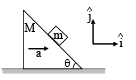Detailed Solution for Test: Force & Newton's Laws of Motion - 2 - Question 2

F. B. D. of block with respect to wedge.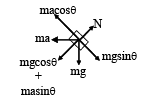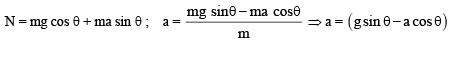Test: Force & Newton's Laws of Motion - 2 - Question 3

### A boy of mass 40 kg is climbing a vertical pole at a constant speed. If the coefficient of friction between his palms and the pole is 0.8 and g = 10 m/s2 the horizontal force that he is applying on the  pole is

Detailed Solution for Test: Force & Newton's Laws of Motion - 2 - Question 3

Here,    μ= 0. 8

Let F be horizontal force that the boy is applying on the pole.
The various forces are acting on the boy as shown in the below figure.
Frictional force.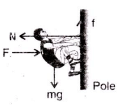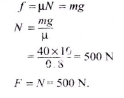Test: Force & Newton's Laws of Motion - 2 - Question 4

In the adjoining figure, the tension in the string connecting A and B is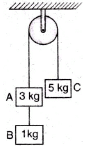Detailed Solution for Test: Force & Newton's Laws of Motion - 2 - Question 4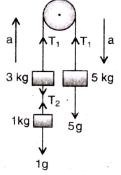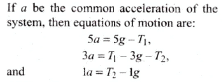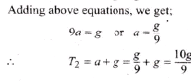Test: Force & Newton's Laws of Motion - 2 - Question 5

In the system shown in the adjoining figure, the acceleration of the 1 kg mass is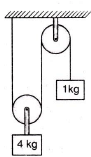Detailed Solution for Test: Force & Newton's Laws of Motion - 2 - Question 5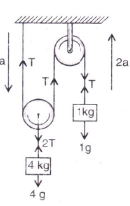Suppose a be the downward acceleration of the 4 kg mass, therefore, 2 a    is the upward acceleration of the I kg mass. Hence, equations of motion are: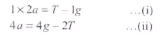Adding, after multiplying the equation (i) by 2.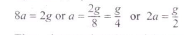Thus, the acceleration of the mass
a 1 kg is g/2 upwards.

Test: Force & Newton's Laws of Motion - 2 - Question 6

Two masses M1 and M2 are attached to the ends of a string which passes over a pulley attached to the top of a double inclined plane of angles of inclination α and β If M2 > M1, the acceleration ‘a’ of the system is given by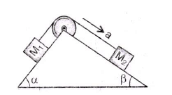Detailed Solution for Test: Force & Newton's Laws of Motion - 2 - Question 6

Suppose M2 moves downwards with an acceleration a and the tension in the string is T.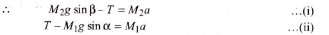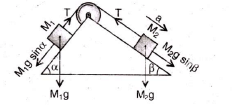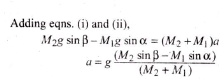Test: Force & Newton's Laws of Motion - 2 - Question 7

Three masses of 1 kg, 6 kg and 3 kg are connected to each other with threads and are placed on a table a as shown in the figure. What is the acceleration with which the system is moving  (Take g = 10 m/s2)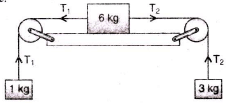Detailed Solution for Test: Force & Newton's Laws of Motion - 2 - Question 7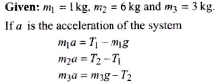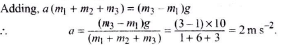Test: Force & Newton's Laws of Motion - 2 - Question 8

The pulley arrangements shown in figure are identical, the mass of the rope being negligible. In case I, the mass m is lifted by attaching a mass 2m to the other end of the rope. In case II, the mass m is lifted by pulling the other end of the rope with a constant downward force, F = 2 mg, where g is acceleration due to gravity. The acceleration of mass m in case I is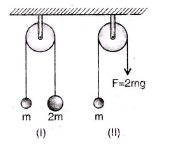Detailed Solution for Test: Force & Newton's Laws of Motion - 2 - Question 8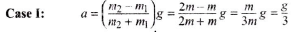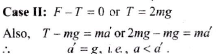Test: Force & Newton's Laws of Motion - 2 - Question 9

In the pulley-block arrangement shown in figure. Find the relation between acceleration of block A and B.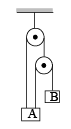Detailed Solution for Test: Force & Newton's Laws of Motion - 2 - Question 9

x1 + x2 = l1

Differentiating with respect to time,  we get v1 + v3 = 0

Again differentiating w.r.t. time,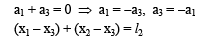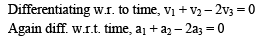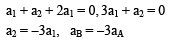Test: Force & Newton's Laws of Motion - 2 - Question 10

Two masses of 1 kg and 5 kg are attached to the ends of a massless string passing over a pulley of negligible weight. The pulley itself is attached to a light spring balance as shown in the figure. The masses start moving during this interval, the reading of spring balance will be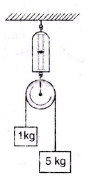Detailed Solution for Test: Force & Newton's Laws of Motion - 2 - Question 10

Applying the 2nd law of motion,
Equating all forces on the string carrying the 2 blocks we get T = 5g/3
The tension in spring balance = 2T
So, the reading is the mass = T/g = 10/3 Kg < 6Kg

Test: Force & Newton's Laws of Motion - 2 - Question 11

Two bodies of mass 4 kg and 6 kg are attached to the ends of a string passing over a pulley. A 4 kg mass is attached to the table top by another string. The tension in this string T1 is equal to (g = 10 m/s2)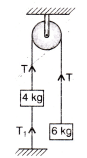Detailed Solution for Test: Force & Newton's Laws of Motion - 2 - Question 11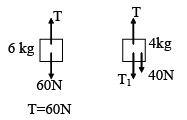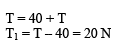Test: Force & Newton's Laws of Motion - 2 - Question 12

Two bodies of mass 6 kg and 4 kg are tied to a string as shown in the adjoining figure. If the table is smooth and pulley frictionless, then acceleration of mass 6 kg will be (g = 10 m/s2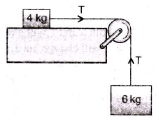Test: Force & Newton's Laws of Motion - 2 - Question 13

Two masses of 8 kg and 4 kg are connected by a string as shown in the figure over a frictionless pulley. The acceleration of the system is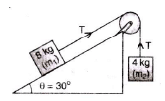Detailed Solution for Test: Force & Newton's Laws of Motion - 2 - Question 13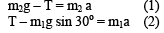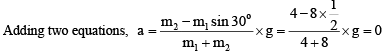Test: Force & Newton's Laws of Motion - 2 - Question 14

A trolley T of mass 5 kg on a horizontal smooth surface is pulled by a load of 2 kg through a uniform rope ABC of length 2 m and mass 1 kg. As the load falls from BC = 0 to BC = 2 m, its acceleration (in m/s2) changes from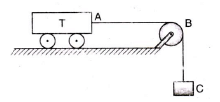Detailed Solution for Test: Force & Newton's Laws of Motion - 2 - Question 14

Initial force = load x g = 2 x 10 = 20 N

∴ initial acceleration = Force/Mass =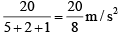Final force = (load + mass of the thread) x g = ( 2 + 1 ) x 10 = 30N

∴  Final acceleration = (30/8) m/s2

Test: Force & Newton's Laws of Motion - 2 - Question 15

Two wooden blocks are moving on a smooth horizontal surface such that the mass m remains stationary with respect to block of mass M as shown in figure. The magnitude of force P is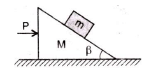Detailed Solution for Test: Force & Newton's Laws of Motion - 2 - Question 15

The difterent torces acting on mass m are shown in the adjoining figure. Acceleration of the system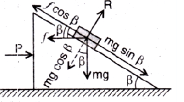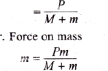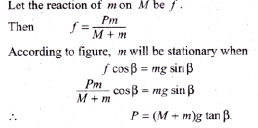Test: Force & Newton's Laws of Motion - 2 - Question 16

A block sliding along inclined plane as shown in figure. If the acceleration of chamber is ‘a’ as shown in the figure. The time required to cover a distance L along inclined plane is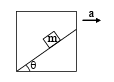Detailed Solution for Test: Force & Newton's Laws of Motion - 2 - Question 16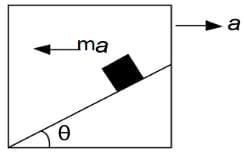Applying pseudo force ma along the left direction of the block.
The net acceleration along the incline will be aeffective​=acosθ+gsinθ
The time required will be L=0×t+½ ​aeffective​t2

⇒ √ 2L/​​ aeffective​
⇒√ 2L /acosθ+gsinθ​​

Test: Force & Newton's Laws of Motion - 2 - Question 17

A block of mass 4 kg is suspended through two light spring balances A and B. Then A and B will read respectively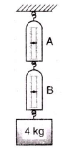Detailed Solution for Test: Force & Newton's Laws of Motion - 2 - Question 17

Tension is uniformly transmitted if the springs are massless.

Test: Force & Newton's Laws of Motion - 2 - Question 18

A light string fixed at one end to a clamp on ground passes over a fixed pulley and hangs at the other side. It makes an angle of 30o with the ground. A monkey of mass 5 kg climbs up the rope. The clamp can tolerate a vertical force of 40 N only. The maximum acceleration in upward direction with which the monkey can climb safely is (neglect friction and take g = 10 m/s2)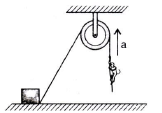Detailed Solution for Test: Force & Newton's Laws of Motion - 2 - Question 18

Let T be the tension in the string.

The upward force exerted on the clamp = T sin 30o = T/2

Given: (T/2) = 40 N   or   T = 80 N

If ‘a’ is the acceleration of the monkey in upward direction,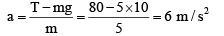Test: Force & Newton's Laws of Motion - 2 - Question 19

If the normal reactional force is doubled, the coefficient of friction is

Detailed Solution for Test: Force & Newton's Laws of Motion - 2 - Question 19

Coefficient of static friction does not depend upon normal reaction.

Test: Force & Newton's Laws of Motion - 2 - Question 20

The force acting on the block pushes it, then pushing of the block will be possible along the surface, if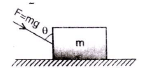Detailed Solution for Test: Force & Newton's Laws of Motion - 2 - Question 20

In this case, vertical component of the force increases the normal reaction, i.e.,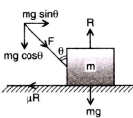R = mg + mg cos θ = mg(1 + cosθ)

Hence, block can be pushed along the horizontal surface when Horizontal component of force > frictional force,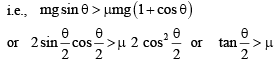Test: Force & Newton's Laws of Motion - 2 - Question 21

An object is placed on the surface of a smooth inclined plane of inclination θ It takes time ‘t’ to reach he bottom. If the same object is allowed to slide down a rough inclined plane of inclination θ it takes time nt to reach the bottom where n is a number greater than 1. The coefficient of friction μ  is given by

Detailed Solution for Test: Force & Newton's Laws of Motion - 2 - Question 21

On smooth inclined plane:
Acceleration of the body = g sin θ If s be the distance travelled, then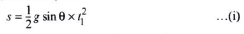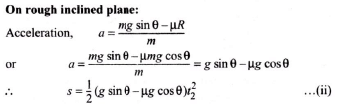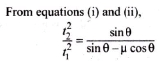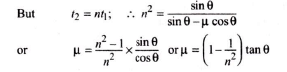Test: Force & Newton's Laws of Motion - 2 - Question 22

The frictional force between two surfaces is independent of

Detailed Solution for Test: Force & Newton's Laws of Motion - 2 - Question 22

The frictional force between two surfaces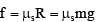where μs depends on the nature of surface and their materials. Thus, frictional force is independent of area of contact of the surfaces.

Test: Force & Newton's Laws of Motion - 2 - Question 23

A body of mass 2 kg rests on a rough inclined plane making an angle 30o with the horizontal. The coefficient of static friction between the block and the plane is 0.7. The frictional force on the block is

Detailed Solution for Test: Force & Newton's Laws of Motion - 2 - Question 23

The body is at rest on the inclined plane, as μ > tan θ  force of friction = mg sin 30o = 2 x 9.8 x (1/2) = 9.8 N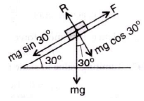Test: Force & Newton's Laws of Motion - 2 - Question 24

A man walks over a rough surface, the angle between the force of friction and the instantaneous velocity of the person is

Detailed Solution for Test: Force & Newton's Laws of Motion - 2 - Question 24

When a man walks on a rough surface, it is the frictional force which is responsible for motion, i.e, required angle between frictional force and instantaneous velocity is zero.

Test: Force & Newton's Laws of Motion - 2 - Question 25

A heavy block of mass M is slowly placed on a conveyor belt moving with a speed v. The coefficient of friction between the block and the belt is μ. Through what distance will the block slide on the belt?

Detailed Solution for Test: Force & Newton's Laws of Motion - 2 - Question 25

Frictional force on the block = μMg

It will be causing acceleration a =μMg/M = μg

The block slide on the ball till its velocity becomes ‘v’ given by the equation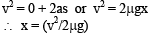Test: Force & Newton's Laws of Motion - 2 - Question 26

A uniform rope of length l lies on a table. If the coefficient of friction is m, then the maximum length l1of the part of this rope which can overhang from the edge of the table without sliding down is

Detailed Solution for Test: Force & Newton's Laws of Motion - 2 - Question 26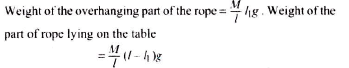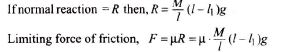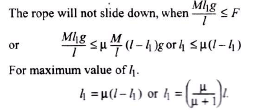Test: Force & Newton's Laws of Motion - 2 - Question 27

A body is moving with uniform velocity of 2 m/s on a rough level surface. The frictional force on it is 10 N. If the body moves with velocity 4 m/s, the force of friction will be

Detailed Solution for Test: Force & Newton's Laws of Motion - 2 - Question 27

Kinetic friction is constant, hence frictional force will remain same (= 10 N).

Test: Force & Newton's Laws of Motion - 2 - Question 28

The coefficient of static friction μ  between block A of mass 2kg and the table as shown in the figure is 0.2. What would be the maximum mass value of block B so that the two blocks do not move? The string and the pulley are assumed to be smooth and massless (g = 10 m/s2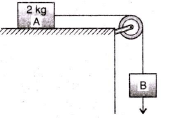Detailed Solution for Test: Force & Newton's Laws of Motion - 2 - Question 28

For the equilibrium,  mBg = μmAg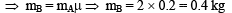Test: Force & Newton's Laws of Motion - 2 - Question 29

At any instant if the velocity of point A is 10 m/s then calculate the velocity of point B?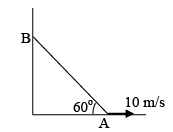Detailed Solution for Test: Force & Newton's Laws of Motion - 2 - Question 29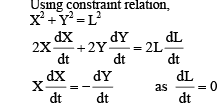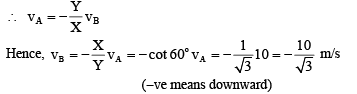Test: Force & Newton's Laws of Motion - 2 - Question 30

Frictional force acting on the block is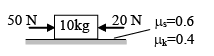Detailed Solution for Test: Force & Newton's Laws of Motion - 2 - Question 30

Net force = 50 - 20 = 30 N
Limiting friction on the body = u (static) × m × g = 0.6 × 10 × 10 = 60 N.
F = 30 N is less than the limiting friction so the body is static. So, a = 0.
Force of friction acting on the body is static friction, f = driving force = 30 N.

## Physics Class 11

127 videos|464 docs|210 tests
 Use Code STAYHOME200 and get INR 200 additional OFF Use Coupon Code
Information about Test: Force & Newton's Laws of Motion - 2 Page
In this test you can find the Exam questions for Test: Force & Newton's Laws of Motion - 2 solved & explained in the simplest way possible. Besides giving Questions and answers for Test: Force & Newton's Laws of Motion - 2, EduRev gives you an ample number of Online tests for practice

## Physics Class 11

127 videos|464 docs|210 tests

### How to Prepare for NEET

Read our guide to prepare for NEET which is created by Toppers & the best Teachers